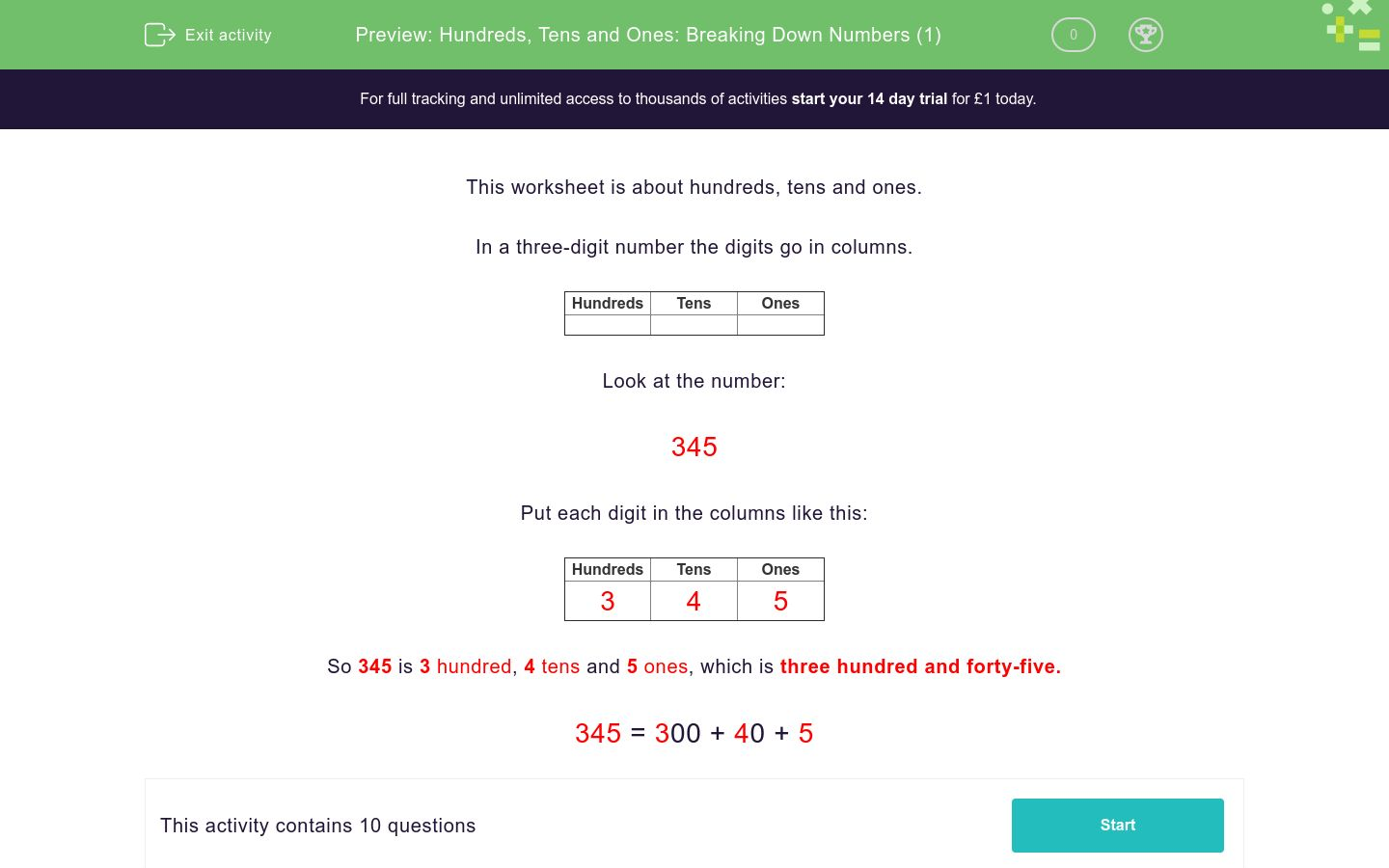# Hundreds, Tens and Ones: Breaking Down Numbers (1)

In this worksheet, students break a number down into its HTO values.Key stage:  KS 2

Curriculum topic:  Number: Number and Place Value

Curriculum subtopic:  Recognise Hundreds, Tens and Ones

Difficulty level:### QUESTION 1 of 10

This worksheet is about hundreds, tens and ones.

In a three-digit number the digits go in columns.

 Hundreds Tens Ones

Look at the number:

345

Put each digit in the columns like this:

 Hundreds Tens Ones 3 4 5

So 345 is 3 hundred, 4 tens and 5 ones, which is three hundred and forty-five.

345 = 300 + 40 + 5

Choose the correct answer.

764 = __00 + __0 + 4

700 + 60 + 4

600 + 70 + 4

7000 + 60 + 4

Choose the correct answer.

639 = __00 + __0 + 9

600 + 30 + 9

900 + 60 + 3

6000 + 30 + 9

Choose the correct answer.

311 = __00 + __0 + 1

3000 + 10 + 1

100 + 30 + 1

300 + 10 + 1

Choose the correct answer.

158 = __00 + __0 + 8

100 + 50 + 8

500 + 10 + 8

1000 + 50 + 8

Choose the correct answer.

805 = __00 + __0 + 5

8000 + 50 + 5

500 + 80 + 5

800 + 5

Choose the correct answer.

880 = __00 + __0 + 0

800 + 80 + 0

8 + 80 + 0

8000 + 80 + 0

Choose the correct answer.

998 = __00 + __0 + 8

9000 + 90 + 8

9 + 90 + 8

900 + 90 + 8

Choose the correct answer.

761 = __00 + __0 + 1

700 + 60 + 1

7 + 60 + 1

7000 + 60 + 1

Choose the correct answer.

957 = __00 + __0 + 7

9000 + 50 + 7

9 + 50 + 7

900 + 50 + 7

Choose the correct answer.

453 = __00 + __0 + 3

400 + 50 + 3

4 + 50 + 3

4000 + 50 + 3

• Question 1

Choose the correct answer.

764 = __00 + __0 + 4

700 + 60 + 4
EDDIE SAYS

7 hundred, 6 tens and 4 ones

• Question 2

Choose the correct answer.

639 = __00 + __0 + 9

600 + 30 + 9
EDDIE SAYS

6 hundred, 3 tens and 9 ones

• Question 3

Choose the correct answer.

311 = __00 + __0 + 1

300 + 10 + 1
EDDIE SAYS

3 hundred, 1 ten and 1 one

• Question 4

Choose the correct answer.

158 = __00 + __0 + 8

100 + 50 + 8
EDDIE SAYS

1 hundred, 5 tens and 8 ones

• Question 5

Choose the correct answer.

805 = __00 + __0 + 5

800 + 5
EDDIE SAYS

8 hundred, 0 tens and 5 ones

• Question 6

Choose the correct answer.

880 = __00 + __0 + 0

800 + 80 + 0
EDDIE SAYS

8 hundred, 8 tens and 0 ones

• Question 7

Choose the correct answer.

998 = __00 + __0 + 8

900 + 90 + 8
EDDIE SAYS

9 hundred, 9 tens and 8 ones

• Question 8

Choose the correct answer.

761 = __00 + __0 + 1

700 + 60 + 1
EDDIE SAYS

7 hundred, 6 tens and 1 one

• Question 9

Choose the correct answer.

957 = __00 + __0 + 7

900 + 50 + 7
EDDIE SAYS

9 hundred, 5 tens and 7 ones

• Question 10

Choose the correct answer.

453 = __00 + __0 + 3

400 + 50 + 3
EDDIE SAYS

4 hundred, 5 tens and 3 ones

---- OR ----

Sign up for a £1 trial so you can track and measure your child's progress on this activity.

### What is EdPlace?

We're your National Curriculum aligned online education content provider helping each child succeed in English, maths and science from year 1 to GCSE. With an EdPlace account you’ll be able to track and measure progress, helping each child achieve their best. We build confidence and attainment by personalising each child’s learning at a level that suits them.

Get startedStart your £1 trial today.
Subscribe from £10/month.